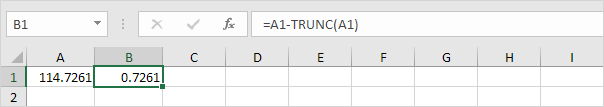# Decimal Places

Learn how to show fewer or more decimal places in Excel without changing the number itself. You can also round a number.

### Decrease Decimal

To decrease the number of decimal places displayed for a cell, simply click the Decrease Decimal button in Excel.

1. For example, enter the value 2.175 into cell A1.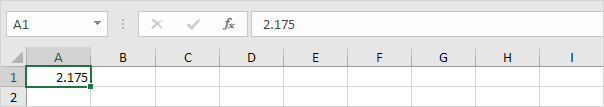2. On the Home tab, in the Number group, click the Decrease Decimal button twice.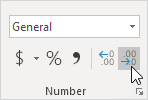Result: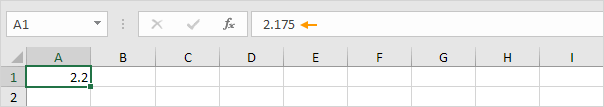Note: if you look at the formula bar, you can see that cell A1 still contains the value 2.175.

3. We can still use this underlying precise value in calculations.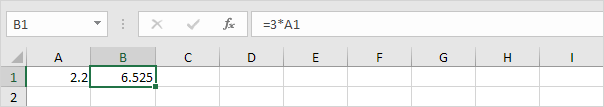Note: if you want to use 2.2 to get a result of 6.6, round the value in cell A1.

### Increase Decimal

To show more decimal places, use the Increase Decimal button in Excel.

1. For example, select the range B1:B5 below.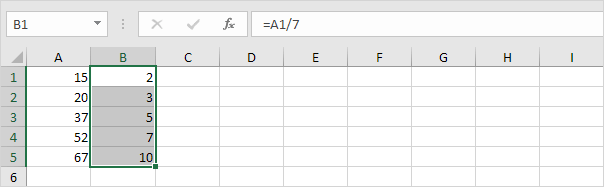Note: these numbers look like integers (whole numbers).

2. On the Home tab, in the Number group, click the Increase Decimal button six times.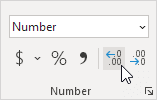Result: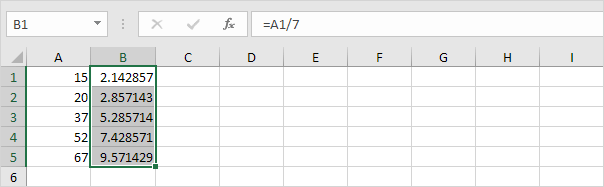Note: Excel stores the exact values of numbers, even if you can't see all the decimal places (except when you're using functions to change the number of decimal places, which we will see next).

### Useful Functions

Excel offers several useful functions to change the number of decimal places of a number.

1. The ROUND function below rounds a number to two decimal places.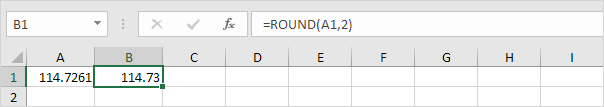Note: if you round a number, you lose precision. For example, if you're using the value in cell B1 in calculations, Excel uses the value 114.73 (not 114.7261).

2. The TRUNC function in Excel removes decimal places from a given number (without rounding).Note: if you use the TRUNC function, you lose precision. Use the second argument to specify how many decimals you want to keep.

3. To extract only the decimal part of a number, use the following formula.